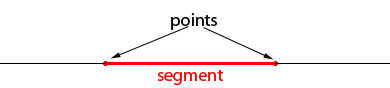SEARCH HOMEMath Central Quandaries & QueriesQuestion from Izsak: What is the formula to find the number of segments that can be named by a given number of points on a line?Hi Izsak,

A line segment is determined by two different points on the line. The segment consists of the two points and all points between them.If you have $n$ points on the line how many ways are there to select two of them for the endpoints of a segment? How many segments are there?

PennyMath Central is supported by the University of Regina and The Pacific Institute for the Mathematical Sciences.